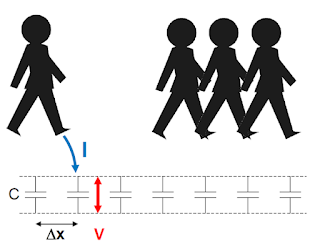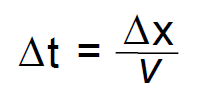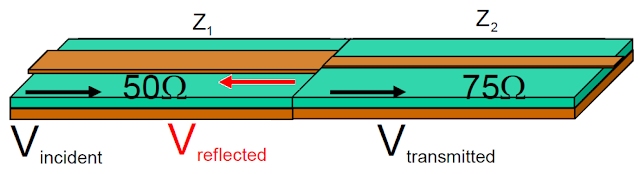## You need to test, we're here to help.

You need to test, we're here to help.

## 31 January 2022

### Transmission Lines for Oscilloscope Users, Part 2Figure 1: A transmission line can be seen asa series of "buckets" of capacitance chargedto a voltage by the signal as it "walks the line."
In Part 1, we experimented with the signal rise time measurement and saw that it appeared to increase substantially as the length of the interconnecting cable was increased. To understand why, we revisited some basic principles of signal integrity:

1. All interconnects are transmission lines.
2. Signals are dynamic, and once launched, cannot be prevented from propagating down the transmission line.

### Be the Signal

To illustrate the dynamic nature of signals, imagine a very simple, 1 ns long, 50 Ω impedance transmission line. As a 1 V signal is launched into the transmission line and propagates, at each step along the way it asks "What's the impedance of the environment?" at its leading edge. That is the instantaneous impedance, notated as Z. Impedance is always defined as the ratio of a voltage to a current. We know the voltage of this signal (1 V), but how do we find the current at the edge?

Let’s consider this transmission line as a series of little buckets of capacitance (Figure 1). If we imagine walking down the transmission line with the signal, at each step we see a capacitance (C) between the signal path and the return path. The amount is the capacitance per length (CL) of the transmission line times the size of our step (Δx).

Before we step on a capacitor, there's zero volts across it. As we step on it, we dump a certain amount of charge (ΔQ) to bring that capacitor up to 1 V, leaving 1 V in our wake. The charge is just the capacitance times the voltage we charge it to.The time it takes per step (Δt) is the distance that we're going between each footstep (Δx) divided by the speed of the signal (v).

If we walk down the line at a constant speed, we dump the same amount of charge in the same amount of time. That's known as constant current (I), calculated as the amount of charge dumped (ΔQ) divided by how long it takes to go between “buckets” (Δt). The current coming out of our foot as we step is the product of our speed (v), the capacitance per length (CL), the size of our footstep (Δx) and the voltage charge (V), divided by the size of our footstep (Δx). As it happens, through the magic of calculus, the size of our footstep doesn't matter—it cancels out.

Since the instantaneous impedance (Z) is the ratio of the voltage we apply (V) and the current (I) charging each “bucket”, now we can calculate what is that instantaneous impedance we see as we step down the transmission line. It's the voltage we applied (V) divided by the product of the speed, capacitance per unit length and the voltage (vCLV), which we calculate here as 1 over the product of the speed of the signal times the capacitance per length (1/vCL).

If we have a transmission line that is uniform, with one value of instantaneous impedance at each step along the way, we say that transmission line can be characterized by that one instantaneous impedance value, known as the characteristic impedance.

Which bring us to our next fundamental principle…

### Principle 3: Any change in instantaneous impedance will cause reflections

Say we have two transmission lines in series, the first with a characteristic impedance of
50 Ω, the second with a characteristic impedance of 75 Ω.Figure 2: A composite transmission line consisting of a section with a 50 Ω characteristic impedance and a second section with a75 Ω characteristic impedance.

The incident signal (Vi) is launched into the 50 Ω section, and as it propagates, it sees 50, 50, 50 then suddenly 75 Ω. As it encounters the 75 Ω impedance, the signal will be distorted, and some energy will reflect back toward the signal source (Vre). The ratio of reflected signal to incident signal is called the reflection coefficient, represented by the Greek letter rho (⍴) and calculated as the second impedance minus the first divided by their sum. The signal that continues in the direction of propagation is the transmitted signal (Vt). Its amplitude is described by the transmission coefficient, (t).

When our 1 V signal encounters the change from 50 to 75 Ω, one-third of a volt will be reflected back toward the source, based on the calculated value of the reflection coefficient. Reflection is a characteristic of the dynamic nature of signals in a transmission line.

One more thing is necessary to solve our rise time measurement problem, and that is to characterize the Cal signal voltage source in order to understand how it reacts with the coaxial cables we used to connect it to the oscilloscope. We’ll pursue that in our next post.

Watch Dr. Eric Bogatin explain these basic principles of signal integrity in our on-demand webinar, What Every Oscilloscope User Needs To know About Transmission Lines.

Ready to go beyond “Transmission Lines 101”? You’ll find “Transmission Lines 201” in our earlier series:

Transmission Lines, Part I

Transmission Lines, Part II